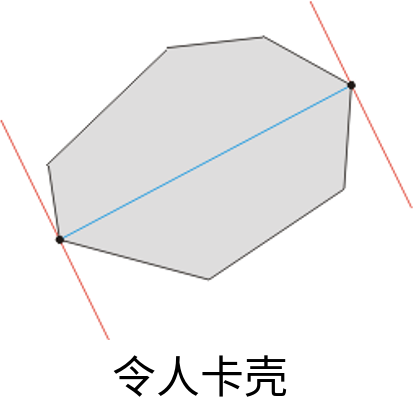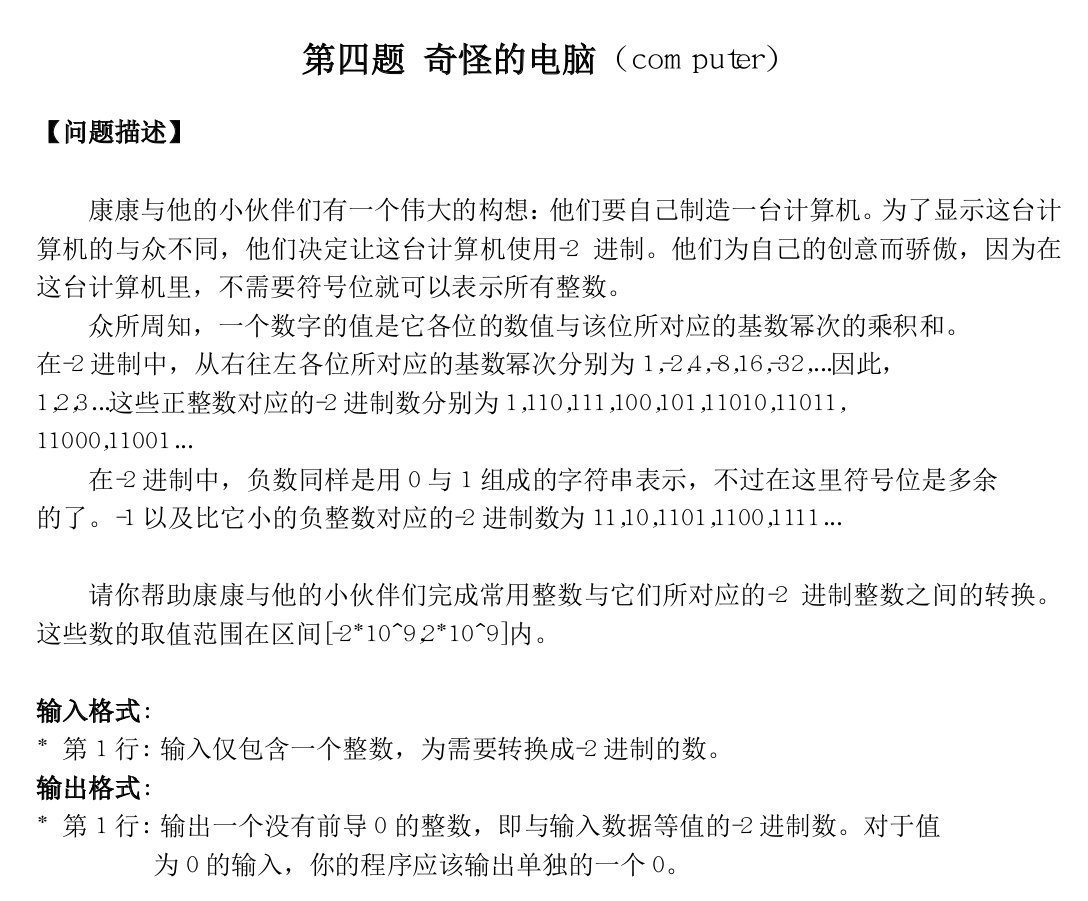-13


110111


• 如果 $B$的下一位为 $1$，那直接把 $A$的当前位设为 $0$，然后让 $B$的下一位也置为 $0$，这样相当于二进制下给 $A$进一位
• 否则把 $B$的下一位置为 $1$，把 $A$的 “下一个位置的下一个位置” 置为 $1$，然后把 $A$的当前位置为 $0$，也相当于进了一位

$B$的同理

（其实我不确定我这个做法是否是最优的）

#include <bits/stdc++.h>

using namespace std;

typedef long long LL;

template<typename _Tp> inline void IN(_Tp& dig)
{
char c; bool flag = 0; dig = 0;
while (c = getchar(), !isdigit(c)) if (c == '-') flag = 1;
while (isdigit(c)) dig = dig * 10 + c - '0', c = getchar();
if (flag) dig = -dig;
}

LL n, m;

bool A, B;

void markA(int), markB(int);

void markA(int a)
{
if (!A[a]) A[a] = 1;
else
{
if (B[a + 1]) B[a + 1] = 0;
else markA(a + 2), markB(a + 1);
A[a] = 0;
}
}

void markB(int a)
{
if (!B[a]) B[a] = 1;
else
{
if (A[a + 1]) A[a + 1] = 0;
else markB(a + 2), markA(a + 1);
B[a] = 0;
}
}

int main(int argc, char const* argv[])
{
IN(n);
if (n < 0)
{
m = 1;
while (m + n < 0) m <<= 1;
n += m;
}
int x = 0;
while (n)
{
if (n & 1)
{
if (x & 1) markA(x + 1), markB(x);
else markA(x);
}
n >>= 1, x++;
}
if (m)
{
x = 0;
while ((1ll << x) < m) x++;
if (x & 1) markB(x);
else markB(x + 1), markA(x);
}
x = 64;
while (!A[x] && !B[x] && x > 0) x--;
while (x >= 0) printf("%d", (int)(A[x] || B[x])), x--;
putchar(10);
return 0;
}


#### Remmina

No puzzle that couldn't be solved.